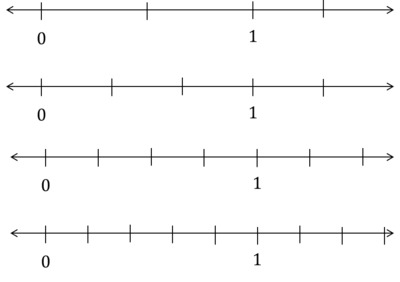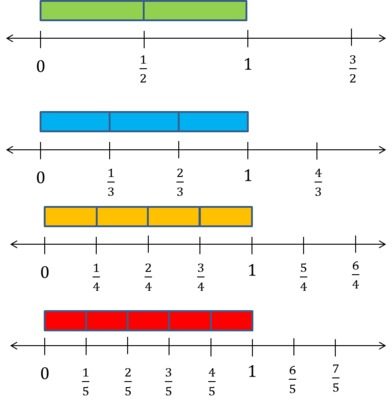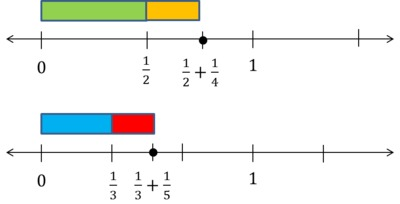# Comparing Sums of Unit Fractions

Alignments to Content Standards: 4.NF.B.3.a

Use <, =, or > to compare the following sums:

1. $\frac12 + \frac14$ ________ $\frac13 + \frac15$
2. $\frac13 + \frac12$ ________ $\frac13 + \frac14$

## IM Commentary

The purpose of this task is to help develop students' understanding of addition of fractions; it is intended as an instructional task. Notice that students are not asked to find the sum because in grade 4, students are limited to computing sums of fractions with like denominators (i.e. fractions where 1 denominator is a multiple of the other). Rather, they need to apply a firm understanding of unit fractions (fractions with one in the numerator) and reason about their relative size. That understanding begins with area models of fractions in grades one and two and expands to the number line in grade 3. With experience, students come to “know” that $\frac14$ is less (smaller) than $\frac13$ because dividing a whole into 4 rather than 3 pieces means there are more pieces, so they must be smaller.

To be successful with this type of problems, students must be able to easily identify the largest (or smallest) fraction in a group of unit fractions.

Struggling students can be given an easier version that repeats one of the fractions in both sums like:

$\frac12 + \frac15$________$\frac12 + \frac14$

Students who can answer the original problem with ease can be asked about differences in problems like this:

$\frac12 – \frac15$________$\frac12 – \frac13$

$\frac12 – \frac15$________$\frac13 – \frac15$

## Solutions

Solution: Compare terms separately

(a) $\frac12$ is greater than $\frac13$ and $\frac14$ is greater than $\frac15$. So

$$\frac12 + \frac14 > \frac13 + \frac15$$

(b) $\frac12$ is greater than $\frac14$, so if I add $\frac13$ to both, I get

$$\frac13 + \frac12 > \frac13 + \frac14$$

Solution: Compare the denominators

(a) By comparing the denominators I know that 1/2 is more than 1/3 because 2 is less than 3. I then compare 1/4 to 1/5 and know that 1/4 is more than 1/5 because 4 is less than 5. The sum of two bigger numbers is greater than the sum of two smaller numbers. $$\frac12 + \frac14 > \frac13 + \frac15$$ (b) I know that 1/2 is more than 1/4 because 2 is less than 4. If I add 1/3 to a smaller number, the result will be less than if I add 1/3 to a bigger number. $$\frac13 + \frac12 > \frac13 + \frac14$$

Solution: Compare visual representations

By fourth grade, students should be able to reason about the relative sizes of unit fractions based on the meaning of the denominators, but for those who need some additional support, the teacher can provide a number line. The issue with student-made number lines is that they may not be drawn precisely, and so the relative sizes may not be apparent. Either students should draw the number lines very carefully, or they should be given number lines with precisely drawn tick marks like those shown below:The number lines should be separated from each other and students should label the tick marks. The colored bars shown below are used to help make the connection between lengths from zero and points on the number line, but the the fractions and their sums should be clearly indicated as points on the number line.(a) First, a student marks of 1/2, 1/3, 1/4, and 1/5 on different number lines. Then they mark a point that is 1/4 to the right of 1/2 and label that 1/2+1/4. Similarly, they should mark a point that is 1/5 to the right of 1/3 and label that 1/3+1/5.$$\frac12 + \frac14 > \frac13 + \frac15$$ They should still articulate the reason why this comparison must be valid. For example, the sum of two bigger numbers is greater than the sum of two smaller numbers. (b) Students can start from 1/3 and part 1/4 and then 1/2 to the right of that.$$\frac13 + \frac12 > \frac13 + \frac14$$ They should still articulate the reason why this comparison must be valid. For example, adding a bigger number makes a greater sum than adding a smaller number.